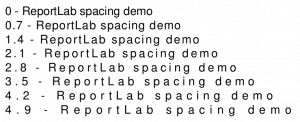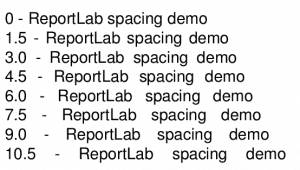# ReportLab 101: The textobject

The ReportLab toolkit provides multiple ways for you to generate text on your PDFs. The most popular examples that I have seen are using canvas methods or using PLATYPUS. The canvas method that you will likely see the most is drawString. Here is an example:

```from reportlab.pdfgen import canvas

c = canvas.Canvas("hello.pdf")
c.drawString(100, 100, "Welcome to Reportlab!")
c.showPage()
c.save()
```

Basically all that does is draw a string at the x/y coordinates given. Using PLATYPUS is significantly more complicated:

```from reportlab.lib.pagesizes import letter
from reportlab.platypus import SimpleDocTemplate, Paragraph
from reportlab.lib.styles import getSampleStyleSheet

def hello():
doc = SimpleDocTemplate("hello_platypus.pdf",
pagesize=letter,
rightMargin=72,
leftMargin=72,
topMargin=72,
bottomMargin=18)
styles = getSampleStyleSheet()

flowables = []

text = "Hello, I'm a Paragraph"
para = Paragraph(text, style=styles["Normal"])
flowables.append(para)

doc.build(flowables)

if __name__ == '__main__':
hello()
```

You will note that most of the time when you use PLATYPUS, you will need to use a template, a style and a paragraph or some other Flowable. But let's go back to the canvas. It actually has another method of generating text that ReportLab calls the textobject. Frankly I have never had the need for one of these as ReportLab's Paragraph class gives you more than enough control over the presentation of your text. But if you depend on using the low level canvas for generating your PDFs, then you will like to know that a textobject will make PDF generation faster because it doesn't use individual calls to drawString.

### The textobject

The best way to learn something new in my experience is to just try and write a minimal demo of some sort. So let's write some code and see how the textobject works:

```# textobject_demo.py

from reportlab.lib import colors
from reportlab.lib.pagesizes import letter
from reportlab.pdfgen import canvas

def textobject_demo():
my_canvas = canvas.Canvas("txt_obj.pdf",
pagesize=letter)
# Create textobject
textobject = my_canvas.beginText()

# Set text location (x, y)
textobject.setTextOrigin(10, 730)

# Set font face and size
textobject.setFont('Times-Roman', 12)

# Write a line of text + carriage return
textobject.textLine(text='Python rocks!')

# Change text color
textobject.setFillColor(colors.red)

# Write red text
textobject.textLine(text='Python rocks in red!')

# Write text to the canvas
my_canvas.drawText(textobject)

my_canvas.save()

if __name__ == '__main__':
textobject_demo()
```

Here we learn that to create a textobject, we need to call the canvas's beginText method. If you happen to print out the textobject, you will find that it's technically an instance of reportlab.pdfgen.textobject.PDFTextObject. Anyway, now that we have a textobject, we can set its cursor position using a call to setTextOrigin. Then we set the font face and size as we saw before. The next new item is the call to textLine, which will allow you to write a string to the buffer plus what is basically a carriage return. The docstring for this method states that it makes the "text cursor moves down", but that amounts to a carriage return in my eyes. There is also a textLines method that allows you to write a multiline string out as well.

The next thing we do is set the font color by calling setFillColor. In this example, we set the the next string of text to a red color. The last step is to call drawText, which will actually draw whatever you have in your textobject. If you skip calling drawText, then your text won't be written out and you may end up with an empty PDF document.

There are a lot of other methods you can call from your textobject. For example, if you want to move your cursor's position somewhere other than the very next line, you can call moveCursor. Let's take a look:

```from reportlab.lib.pagesizes import letter
from reportlab.pdfgen import canvas

def textobject_cursor():
canvas_obj = canvas.Canvas("textobj_cursor.pdf", pagesize=letter)

# Create textobject
textobject = canvas_obj.beginText()

# Set text location (x, y)
textobject.setTextOrigin(10, 730)

for indent in range(4):
textobject.textLine('ReportLab cursor demo')
textobject.moveCursor(15, 15)

canvas_obj.drawText(textobject)
canvas_obj.save()

if __name__ == '__main__':
textobject_cursor()
```

Here we just set up a loop that will print out the same string four times, but at four different positions. You will note that we move the cursor 15 points to the right and 15 points down the page with each iteration of the loop. Yes, when using a textobject, a positive y number will move you down.

Now, let's say you would like to change the inter-character spacing; all you need to do is call setCharSpace. In fact, you can do a lot of interesting spacing tricks with textobject, such as changing the space between word using setWordSpace or the space between lines by calling setLeading. Let's take a look at how we might change the spacing of our text:

```from reportlab.lib.pagesizes import letter
from reportlab.pdfgen import canvas

def textobject_char_spacing():
canvas_obj = canvas.Canvas("textobj_char_spacing.pdf",
pagesize=letter)

# Create textobject
textobject = canvas_obj.beginText()

# Set text location (x, y)
textobject.setTextOrigin(10, 730)

spacing = 0
for indent in range(8):
textobject.setCharSpace(spacing)
line = '{} - ReportLab spacing demo'.format(spacing)
textobject.textLine(line)
spacing += 0.7

canvas_obj.drawText(textobject)
canvas_obj.save()

if __name__ == '__main__':
textobject_char_spacing()
```

In this example, we increase the loop factor to 8 iterations and call setCharSpace() each time through the loop. We start with zero spacing and then add 0.7 in each iteration. You can see the result here:Now let's see how applying word spacing effects our text:

```from reportlab.lib.pagesizes import letter
from reportlab.pdfgen import canvas

def wordspacer():
canvas_obj = canvas.Canvas("textobj_word_spacing.pdf",
pagesize=letter)

# Create textobject
textobject = canvas_obj.beginText()

# Set text location (x, y)
textobject.setTextOrigin(10, 730)

word_spacing = 0
for indent in range(8):
textobject.setWordSpace(word_spacing)
line = '{} - ReportLab spacing demo'.format(word_spacing)
textobject.textLine(line)
word_spacing += 1.5

canvas_obj.drawText(textobject)
canvas_obj.save()

if __name__ == '__main__':
wordspacer()
```

This example is pretty much the same as the previous one, but you will note that we are calling setWordSpace() instead of setCharSpace() and we are increasing the spacing by a factor of 1.5 in this example. The resulting text looks like this:If you would like to create a superscript or subscript, then you would want to call **setRise** on your textobject. Let's create a demo that demonstrates how setting the rise works in ReportLab:

```from reportlab.lib.pagesizes import letter
from reportlab.pdfgen import canvas

def apply_scripting(textobject, text, rise):
textobject.setFont("Helvetica-Oblique", 8)
textobject.setRise(rise)
textobject.textOut(text)
textobject.setFont("Helvetica-Oblique", 12)
textobject.setRise(0)

def main():
canvas_obj = canvas.Canvas("textobj_rising.pdf",
pagesize=letter)

# Create textobject
textobject = canvas_obj.beginText()
textobject.setFont("Helvetica-Oblique", 12)

# Set text location (x, y)
textobject.setTextOrigin(10, 730)

textobject.textOut('ReportLab ')
apply_scripting(textobject, 'superscript ', 7)

textobject.textOut('and ')

apply_scripting(textobject, 'subscript ', -7)

canvas_obj.drawText(textobject)
canvas_obj.save()

if __name__ == '__main__':
main()
```

Here we create a couple of functions, apply_scripting and main. The main function will create our canvas and all the other bits and pieces we need. Then we write out some normal text. The next few lines are where we apply superscripting (positive) and subscripting (negative). Note that we need to set the rise back to zero between the superscript and subscript to make the word, "and", appear in the right location. As soon as you apply a rising value, it will continue to apply from that point on. So you will want to reset it to zero to make sure the text stays in a normal location. You will also note that we set the font size for the super and subscripts to be smaller than the regular text. Here is the result of running this example:Check out ReportLab's user guide for more interesting things you can do or check the source code itself.

### Wrapping Up

We covered a lot of information in this tutorial, but you should now have a pretty good understanding of how you might use ReportLab's textobject on your Canvas. It's super helpful and allows for a lot of easy formatting of your text. Another great benefit is that it's also faster than making multiple drawText() calls. Be sure to give it a try if you get a chance!

Note: This tutorial is based on a section from my latest book, ReportLab: PDF Processing with Python